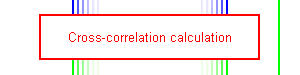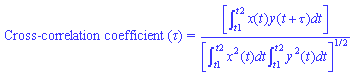# Cross-correlation calculationThe central segment of the measurement model uses a series of cross-correlation calculations as shown in the equation below. This function essentially measures the similarity of two signals.• where x and y are the two signals whose correlation is to be measured
• t1 and t2 are the period over which the correlation is measured
• and τ is an offset between the two signals under measurement
• (adapted from [Ifeachor and Jervis 1993])

This equation may be applied to any two signals, though for the purpose of our measurement model it is employed to analyse a pair of binaural signals (the signals that reach the ears of a listener or a head and torso simulator as discussed previously). In this case, τ is usually measured over a range that is large enough to encompass the maximum interaural time difference that is caused by the physical separation of human ears, typically ±1 ms, though this can be specified in the measurement model.

The final output value is then commonly taken to be the maximum absolute value across the range of τ. However, we tend to use the maximum value, not the maximum absolute value for two reasons. Firstly, we believe that positive and negative output values relate to different perceived attributes and using the absolute value causes this difference to be lost. Secondly, the use of half-wave rectification as described previously causes the range of results from the measurement to be between 0 and 1, meaning that the absolute and raw value maxima will be identical.

Also useful is the fact that the value of τ of the maxima relates to the interaural time difference of the sound. This can be used to predict the perceived location of the sound, though is not a full model of the perceptual process of localisation.

Both the maximum value of the calculation and the related value of τ are passed on for further processing in the measurement model.